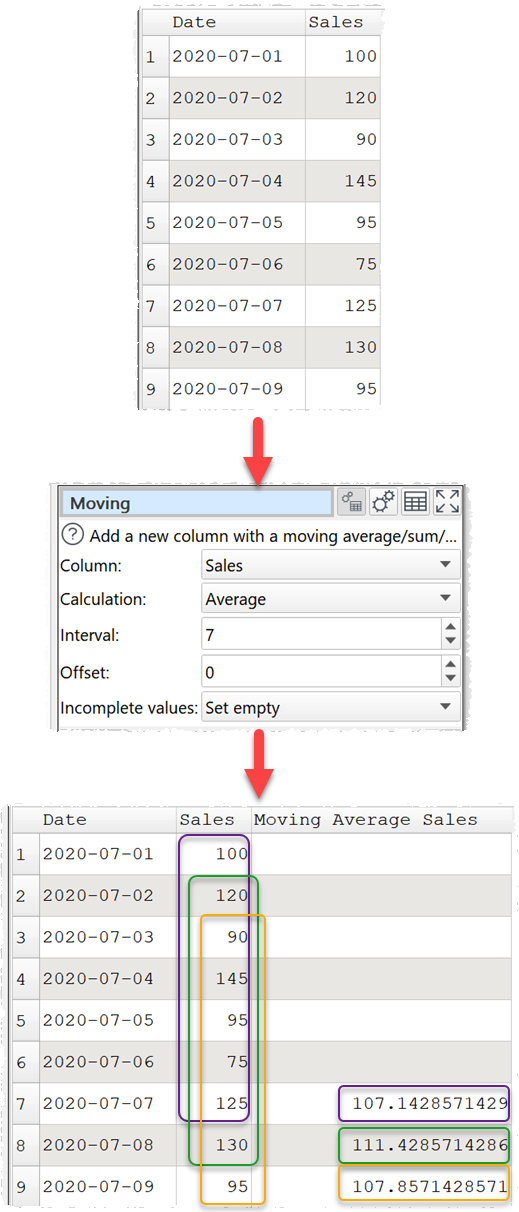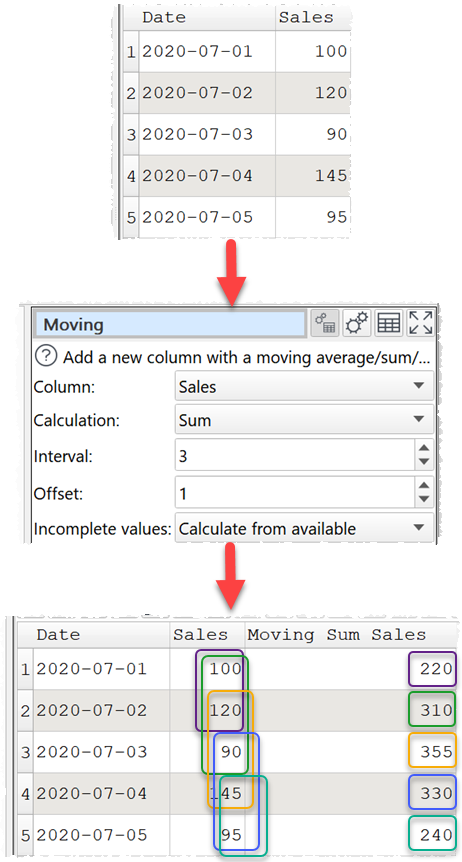# How to calculate a moving average

A simple moving average (SMA) is calculated by taking the average (arithmetic mean) of a set of numeric data values over an interval (e.g. 28 days). It is useful for smoothing out short-term fluctuations and identifying trends in data, such as seasonal cycles. It is often used in technical analysis of financial markets. It is sometimes called a rolling average.

Easy Data Transform can easily calculate a moving average for a column of data over any interval using the Moving transform. For example, calculating the 7 day moving average for some sales data, from 6 days before each date to the current date:Typically the arithmetic mean is calculated over a moving interval. But it is also possible to calculate the median, sum, minimum or maximum over a moving interval. You can also offset the interval so that it doesn’t end at the current value. And you can decide whether to calculate or leave blank values for which you don’t have a full interval of data.See the video above for more details.

This is only one of the many transforms available in Easy Data Transform. It can also help with merging, cleaning, filtering, enriching and reshaping your data. All without coding. You can download the free trial and a few clicks and try it yourself.

### Try it free now!

v1.43.0 for Windows 11 / 10 / 8 / 7 (46 MB)

v1.43.0 for Mac 13.x to 10.13 (78 MB)

Questions or problems?